Successfully reported this slideshow.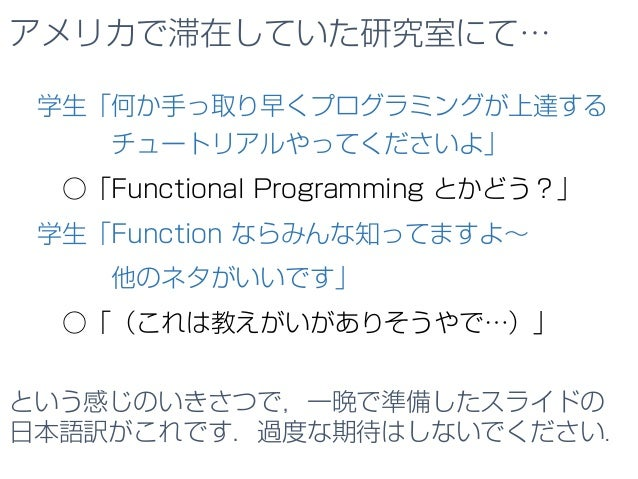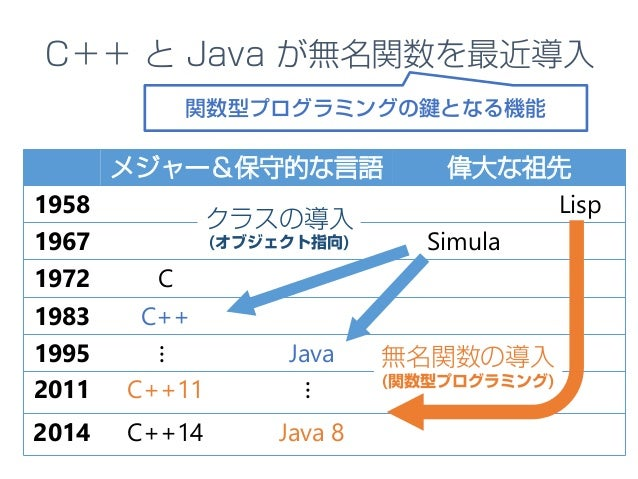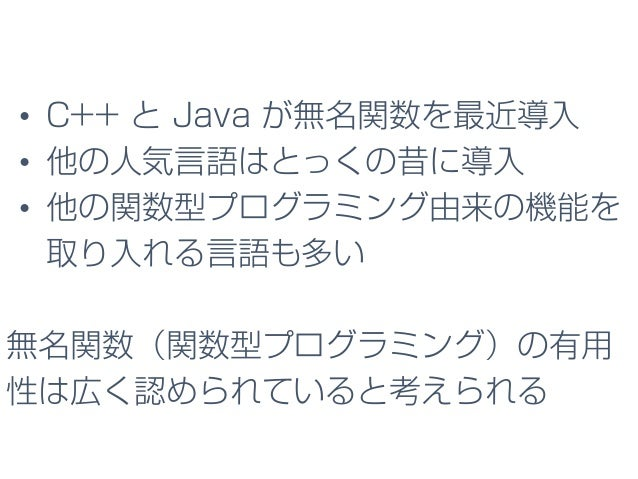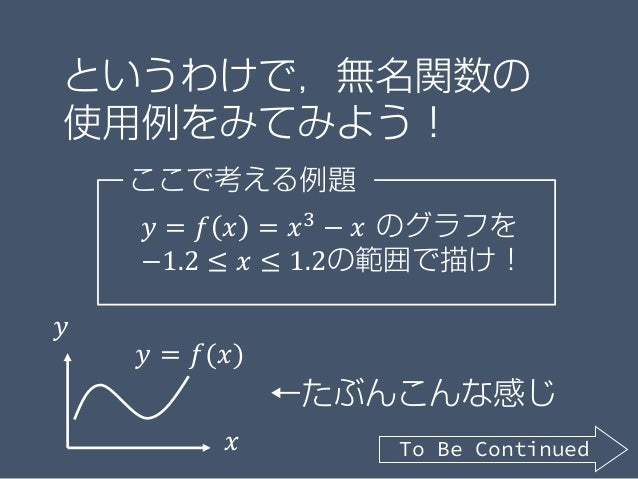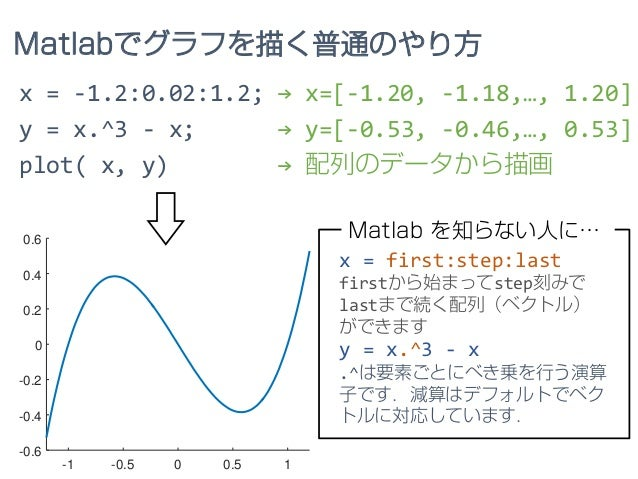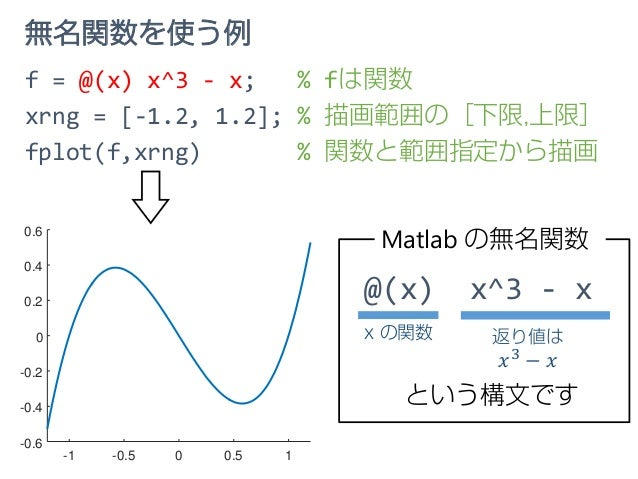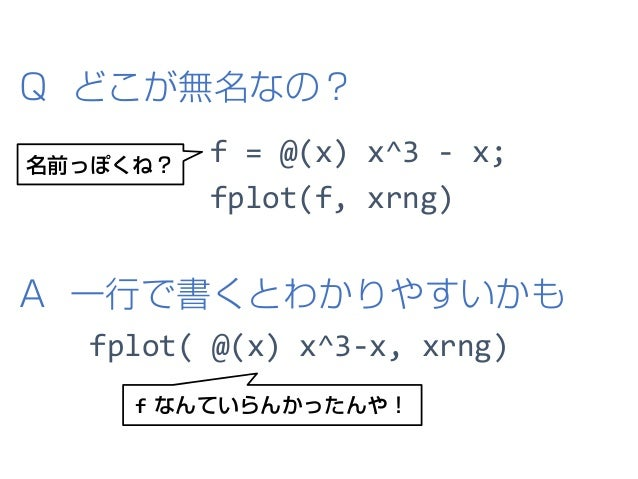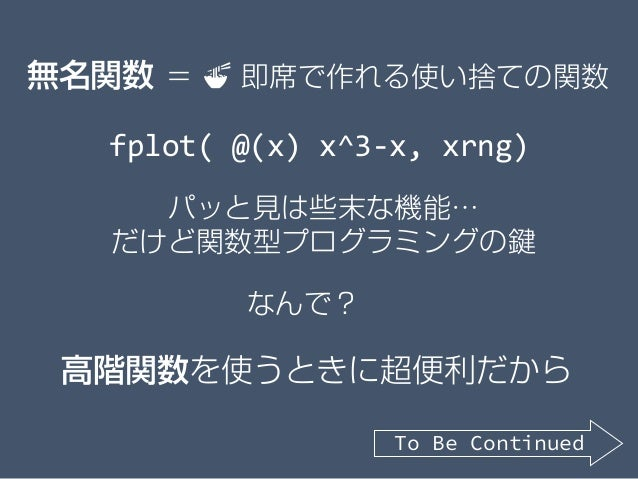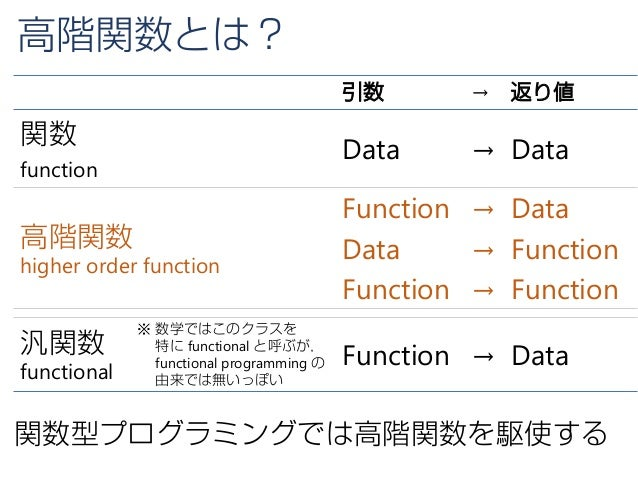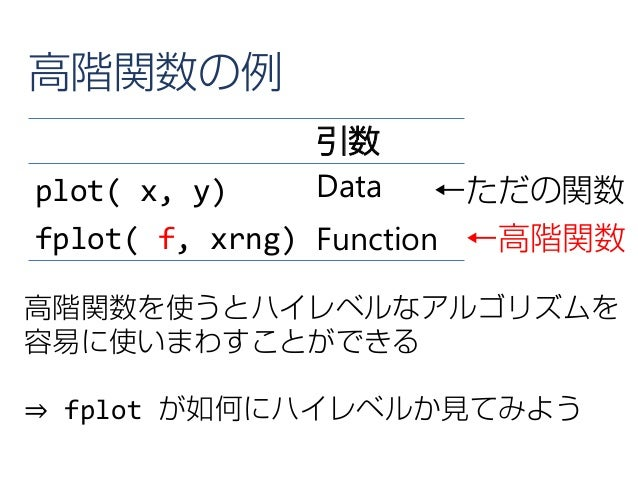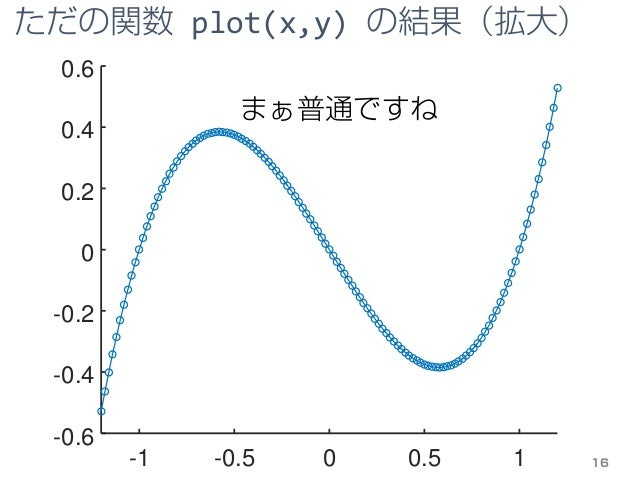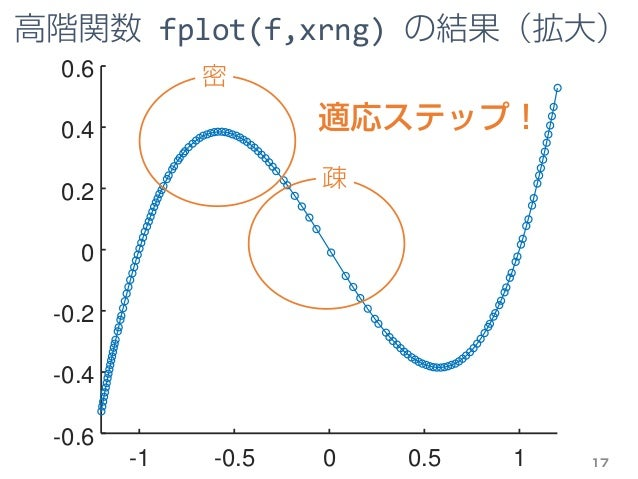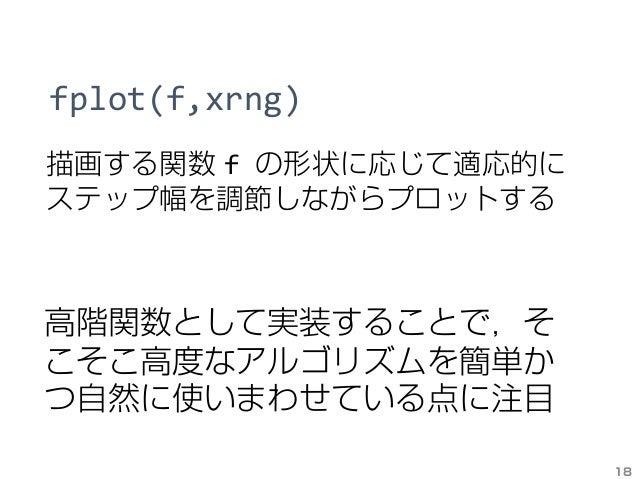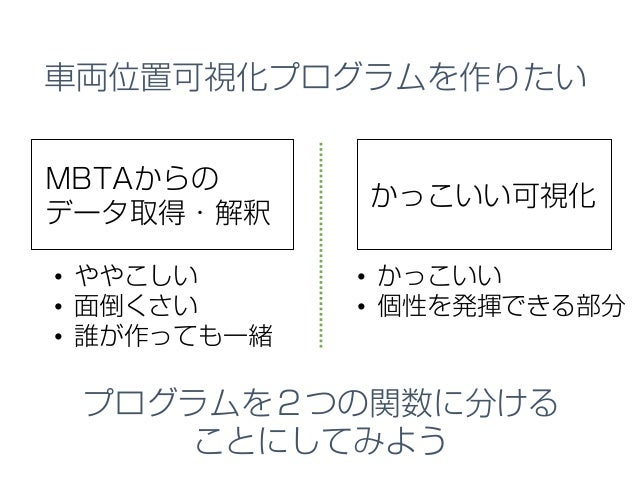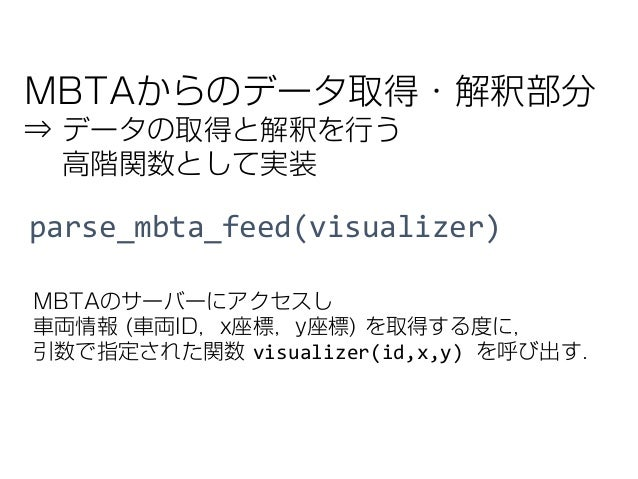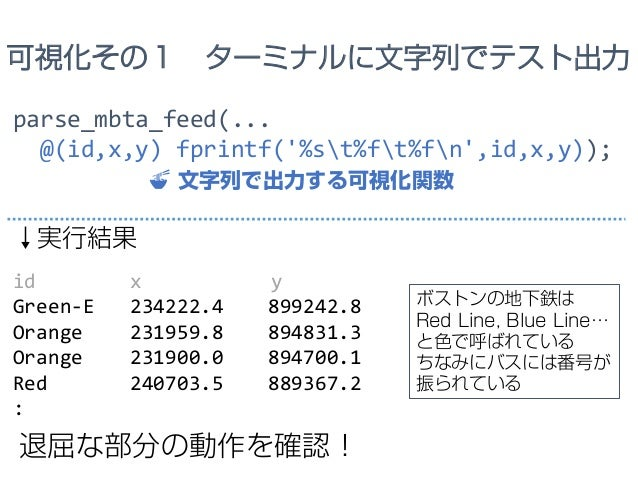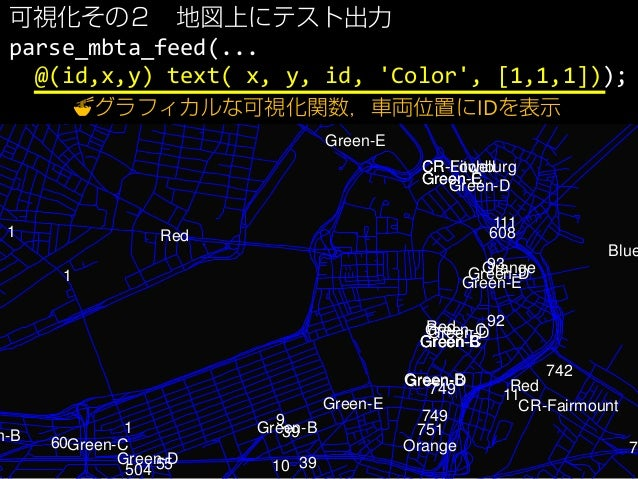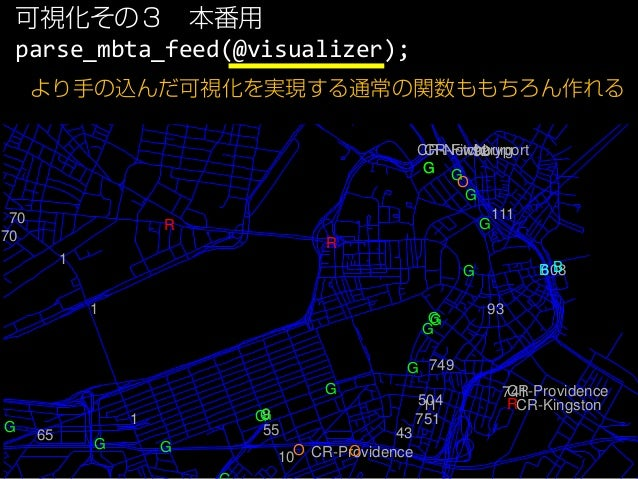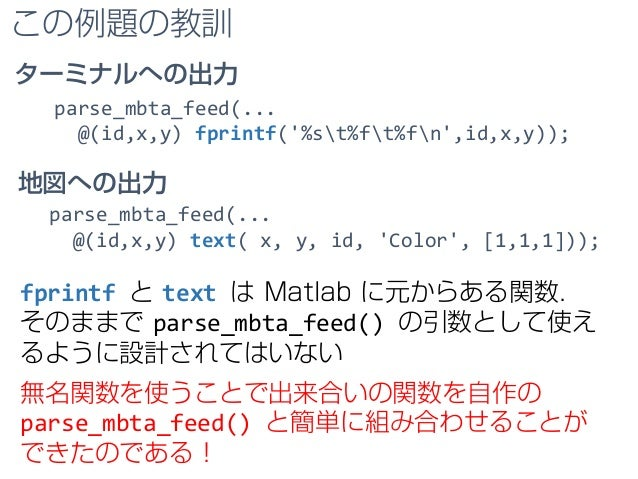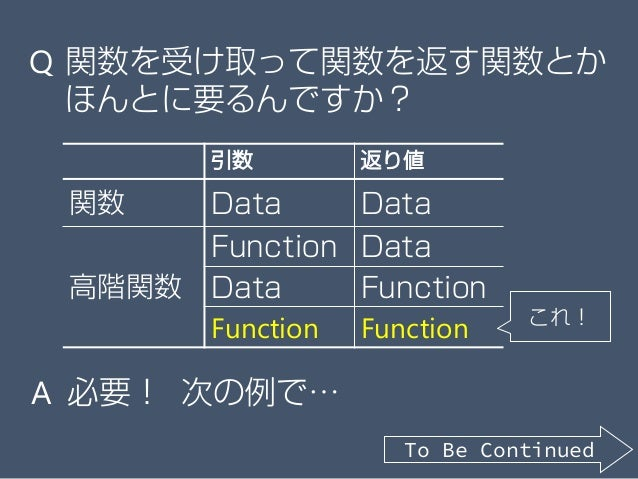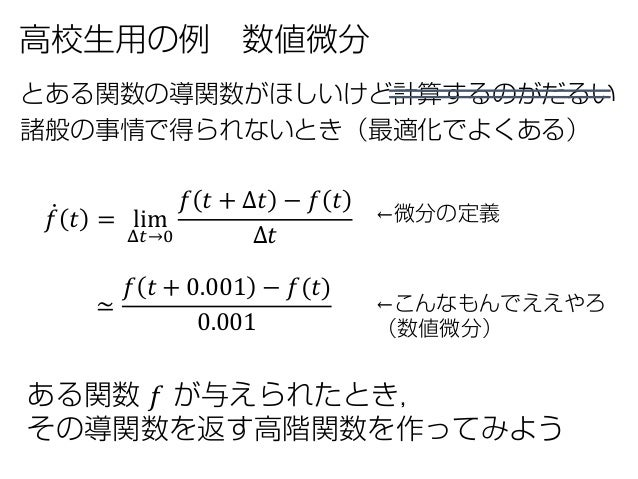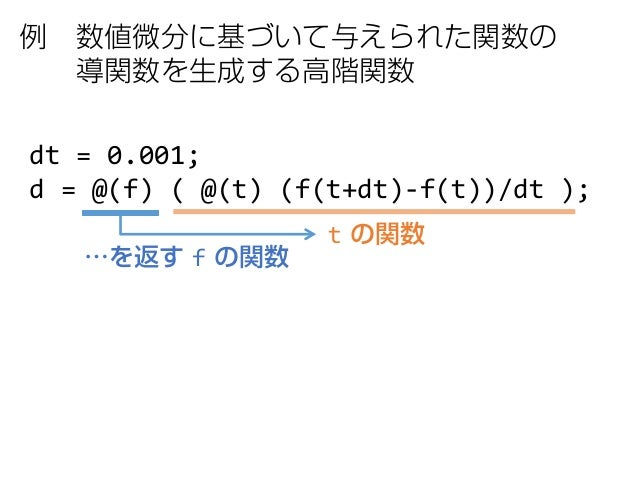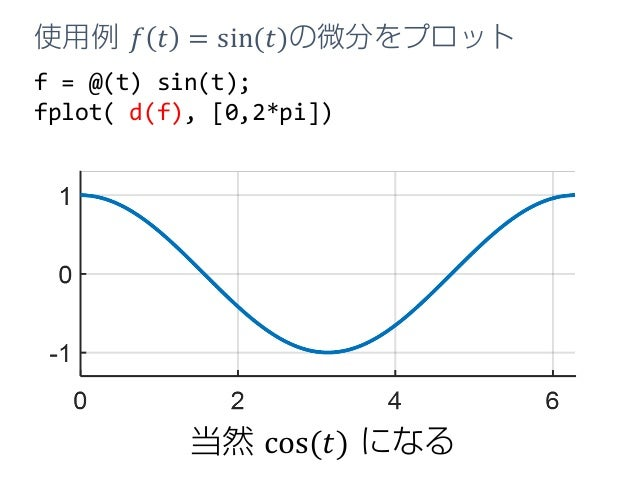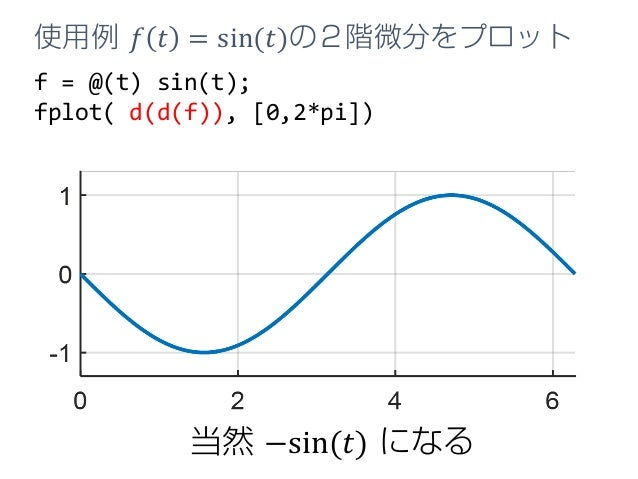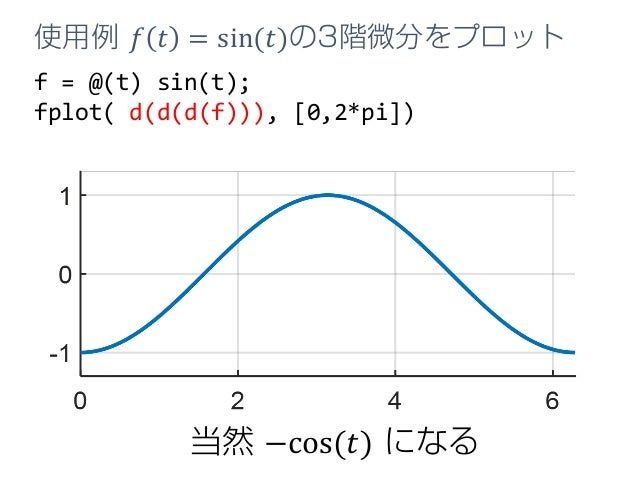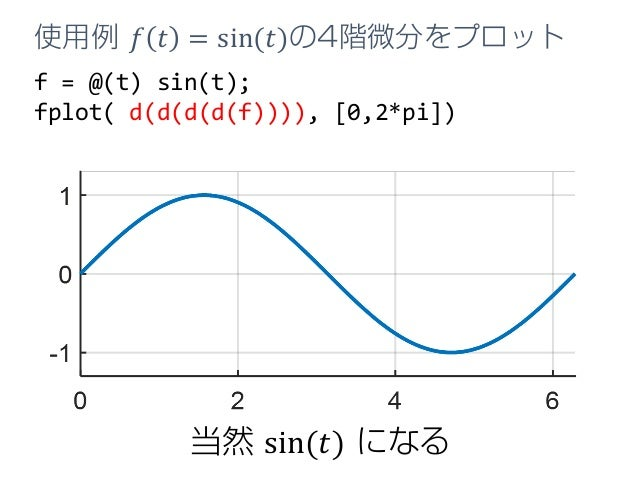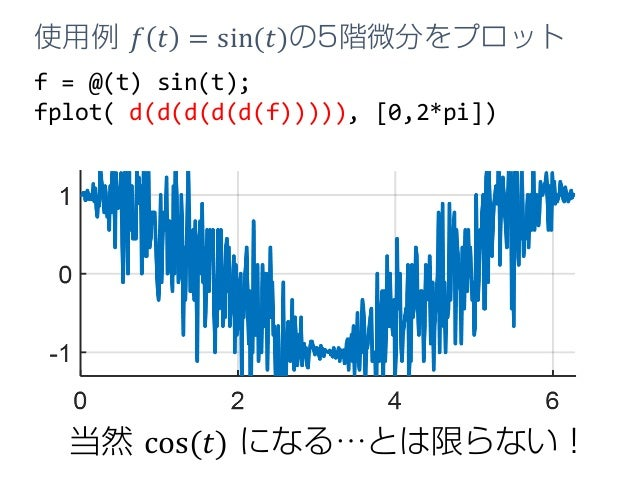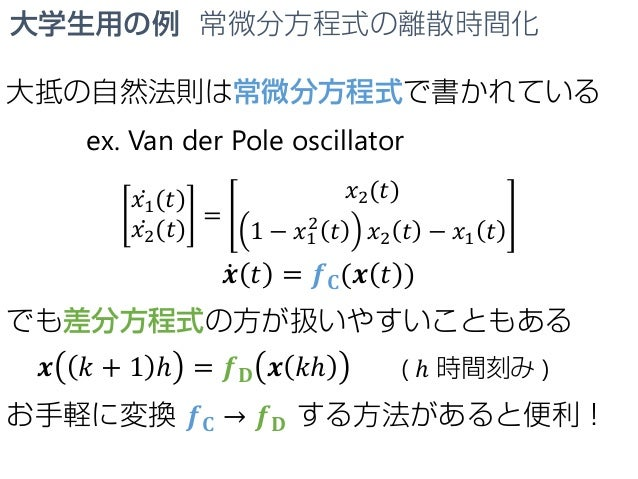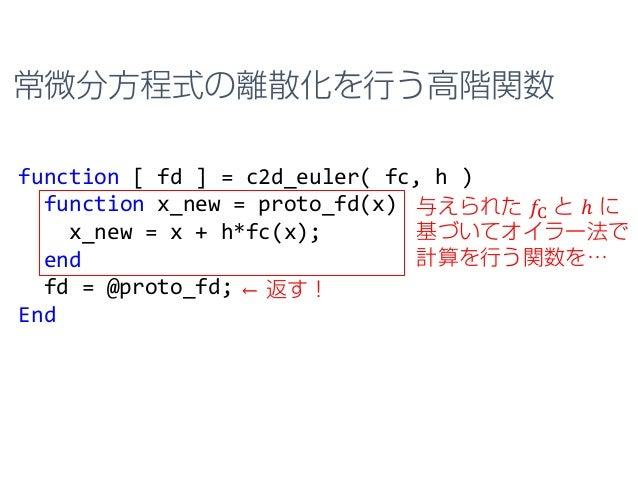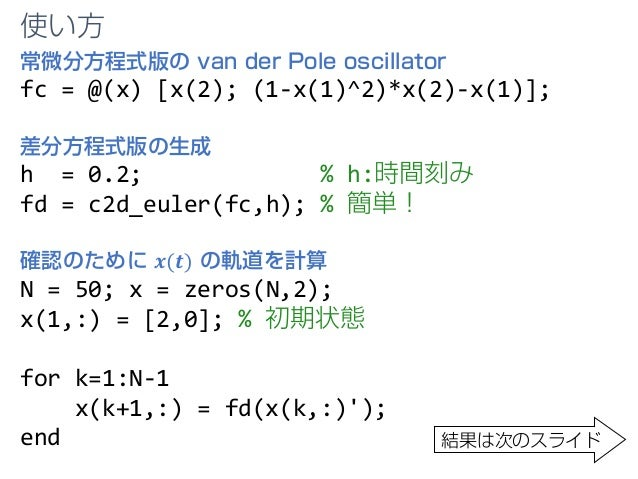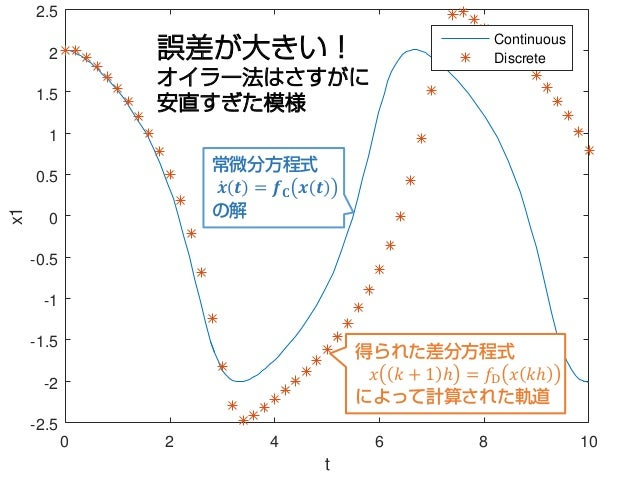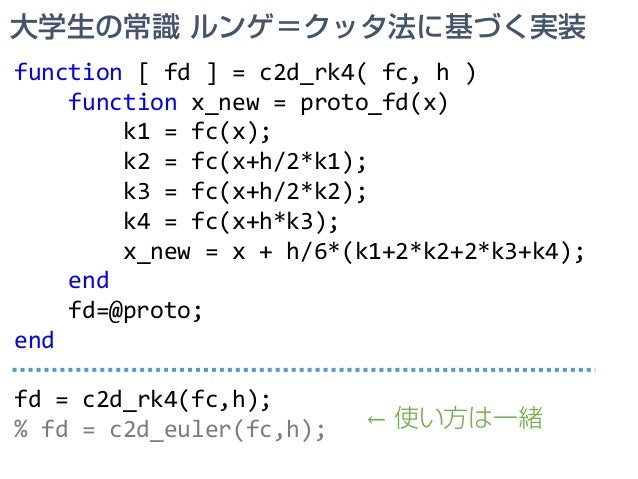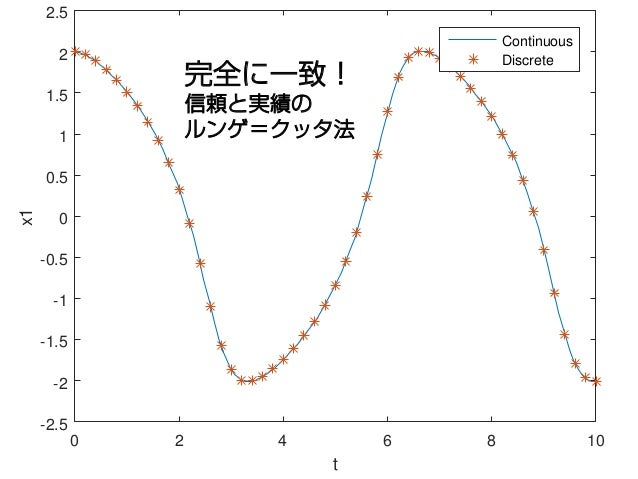Upcoming SlideShare
×

# 関数型プログラミング入門 for Matlab ユーザー

• Full Name
Comment goes here.

Are you sure you want to Yes No• Be the first to comment

### 関数型プログラミング入門 for Matlab ユーザー

1. 1. 関数型プログラミング入門 for Matlabユーザー 丸田 一郎
2. 2. アメリカで滞在していた研究室にて… 学生「何か手っ取り早くプログラミングが上達する チュートリアルやってくださいよ」 ○「Functional Programming とかどう？」 学生「Function ならみんな知ってますよ～ 他のネタがいいです」 ○「（これは教えがいがありそうやで…）」 という感じのいきさつで，一晩で準備したスライドの 日本語訳がこれです．過度な期待はしないでください．
3. 3. このチュートリアルのねらい 対象 • Matlab初級者の大学生/大学院生/研究者 目標 • 関数型プログラミングをちょろっと勉強して 日々のコーディングを効率的にする
4. 4. 関数型プログラミング(FP)って何？ 「関数を組み合わせて処理を記述する」 プログラミングパラダイムです このチュートリアルでは， 「関数を組み合わせて処理を記述する」 うえで有用な機能・テクニックと，その メリットについて例を挙げて説明します． 最初のトピックは「無名関数」です
5. 5. そもそも無名関数（とかFP）って 勉強する価値あるの？ 高確率でペイすると思います その根拠は… Q A To Be Continued
6. 6. メジャー＆保守的な言語 偉大な祖先 1958 Lisp 1967 Simula 1972 C 1983 C++ 1995 ⋮ Java 2011 C++11 ⋮ 2014 C++14 Java 8 クラスの導入 (オブジェクト指向) 無名関数の導入 (関数型プログラミング) C＋＋ と Java が無名関数を最近導入 関数型プログラミングの鍵となる機能
7. 7. • C++ と Java が無名関数を最近導入 • 他の人気言語はとっくの昔に導入 • 他の関数型プログラミング由来の機能を 取り入れる言語も多い 無名関数（関数型プログラミング）の有用 性は広く認められていると考えられる
8. 8. というわけで，無名関数の 使用例をみてみよう！ ここで考える例題 𝑦𝑦 = 𝑓𝑓 𝑥𝑥 = 𝑥𝑥3 − 𝑥𝑥 のグラフを −1.2 ≤ 𝑥𝑥 ≤ 1.2の範囲で描け！ 𝑥𝑥 𝑦𝑦 𝑦𝑦 = 𝑓𝑓(𝑥𝑥) ←たぶんこんな感じ To Be Continued
9. 9. -1 -0.5 0 0.5 1 -0.6 -0.4 -0.2 0 0.2 0.4 0.6 x = -1.2:0.02:1.2; → x=[-1.20, -1.18,…, 1.20] y = x.^3 - x; → y=[-0.53, -0.46,…, 0.53] plot( x, y) → 配列のデータから描画 Matlabでグラフを描く普通のやり方 x = first:step:last firstから始まってstep刻みで lastまで続く配列（ベクトル） ができます y = x.^3 - x .^は要素ごとにべき乗を行う演算 子です．減算はデフォルトでベク トルに対応しています． Matlab を知らない人に…
10. 10. -1 -0.5 0 0.5 1 -0.6 -0.4 -0.2 0 0.2 0.4 0.6 無名関数を使う例 f = @(x) x^3 - x; % fは関数 xrng = [-1.2, 1.2]; % 描画範囲の［下限,上限］ fplot(f,xrng) % 関数と範囲指定から描画 @(x) x^3 - x Matlab の無名関数 x の関数 返り値は 𝑥𝑥3 − 𝑥𝑥 という構文です
11. 11. f = @(x) x^3 - x; fplot(f, xrng) A 一行で書くとわかりやすいかも f なんていらんかったんや！ Q どこが無名なの？ fplot( @(x) x^3-x, xrng) 名前っぽくね？
12. 12. パッと見は些末な機能… だけど関数型プログラミングの鍵 高階関数を使うときに超便利だから 無名関数 ＝ 🍜🍜 即席で作れる使い捨ての関数 fplot( @(x) x^3-x, xrng) なんで？ To Be Continued
13. 13. 高階関数とは？ 引数 → 返り値 関数 function Data → Data 高階関数 higher order function Function → Data Data → Function Function → Function 汎関数 functional ※ 数学ではこのクラスを 特に functional と呼ぶが， functional programming の 由来では無いっぽい Function → Data 関数型プログラミングでは高階関数を駆使する
14. 14. 引数 plot( x, y) Data fplot( f, xrng) Function 高階関数を使うとハイレベルなアルゴリズムを 容易に使いまわすことができる ⇒ fplot が如何にハイレベルか見てみよう ←高階関数 高階関数の例 ←ただの関数
15. 15. -1 -0.5 0 0.5 1 -0.6 -0.4 -0.2 0 0.2 0.4 0.6 ただの関数 plot(x,y) の結果（拡大） 16 まぁ普通ですね
16. 16. -1 -0.5 0 0.5 1 -0.6 -0.4 -0.2 0 0.2 0.4 0.6 高階関数 fplot(f,xrng) の結果（拡大） 17 疎 密 適応ステップ！
17. 17. 18 描画する関数 f の形状に応じて適応的に ステップ幅を調節しながらプロットする fplot(f,xrng) 高階関数として実装することで，そ こそこ高度なアルゴリズムを簡単か つ自然に使いまわせている点に注目
18. 18. まとめ (3/5) 関数型プログラミング  関数を組み合わせて処理を記述するプログラミング パラダイム 無名関数  即席で作れる使い捨ての関数 高階関数  引数や返値として関数をとる関数  高度なアルゴリズムを再利用容易な形で実装する際 に便利 New
19. 19. 一見地味な無名関数だが 高階関数を使う上では必要不可欠 例題を通して確認 To Be Continued
20. 20. 例題 マサチューセッツ湾交通局(MBTA)提供 リアルタイムデータの可視化 バス・電車の位置情報を リアルタイムで提供！
21. 21. 車両位置可視化プログラムを作りたい MBTAからの データ取得・解釈 かっこいい可視化 • ややこしい • 面倒くさい • 誰が作っても一緒 プログラムを２つの関数に分ける ことにしてみよう • かっこいい • 個性を発揮できる部分
22. 22. parse_mbta_feed(visualizer) MBTAからのデータ取得・解釈部分 ⇒ データの取得と解釈を行う 高階関数として実装 MBTAのサーバーにアクセスし 車両情報 (車両ID，x座標，y座標) を取得する度に， 引数で指定された関数 visualizer(id,x,y) を呼び出す．
23. 23. id x y Green-E 234222.4 899242.8 Orange 231959.8 894831.3 Orange 231900.0 894700.1 Red 240703.5 889367.2 : parse_mbta_feed(... @(id,x,y) fprintf('%st%ft%fn',id,x,y)); 🍜🍜 文字列で出力する可視化関数 可視化その１ ターミナルに文字列でテスト出力 ボストンの地下鉄は Red Line, Blue Line… と色で呼ばれている ちなみにバスには番号が 振られている ↓実行結果 退屈な部分の動作を確認！
24. 24. 1 1 1 10 11 111 39 39 504 55 60 608 69 7 742 749 749 751 88 9 92 93 Blue CR-Fairmount CR-FitchburgCR-Lowell Green-B Green-B Green-B n-B Green-B Green-C Green-C Green-C Green-C Green-D Green-D Green-D Green-D Green-D Green-E Green-E Green-E Green-E Green-E Orange Orange Orange Red Red Red 可視化その２ 地図上にテスト出力 parse_mbta_feed(... @(id,x,y) text( x, y, id, 'Color', [1,1,1])); 🍜🍜グラフィカルな可視化関数，車両位置にIDを表示
25. 25. 1 1 1 10 11 111 111 43 504 55 608 65 9 70 70 741 749 751 83 88 9 92 93 BB CR-Fitchburg CR-Kingston CR-Lowell CR-Newburyport CR-Providence CR-Providence G G G G G G G G G G G G G G G G G G O O O O R R R 可視化その３ 本番用 parse_mbta_feed(@visualizer); より手の込んだ可視化を実現する通常の関数ももちろん作れる
26. 26. この例題の教訓 parse_mbta_feed(... @(id,x,y) fprintf('%st%ft%fn',id,x,y)); fprintf と text は Matlab に元からある関数． そのままで parse_mbta_feed() の引数として使え るように設計されてはいない 無名関数を使うことで出来合いの関数を自作の parse_mbta_feed() と簡単に組み合わせることが できたのである！ parse_mbta_feed(... @(id,x,y) text( x, y, id, 'Color', [1,1,1])); ターミナルへの出力 地図への出力
27. 27. まとめ (4/5) 関数型プログラミング  関数を組み合わせて処理を記述するプログラミング パラダイム 無名関数  即席で作れる使い捨ての関数  高階関数を組み合わせる際に便利 高階関数  引数や返値として関数をとる関数  高度なアルゴリズムを再利用容易な形で実装する際 に便利 New
28. 28. 引数 返り値 関数 Data Data 高階関数 Function Data Data Function Function Function Q 関数を受け取って関数を返す関数とか ほんとに要るんですか？ これ！ A 必要！ 次の例で… To Be Continued
29. 29. 高校生用の例 数値微分 とある関数の導関数がほしいけど計算するのがだるい 諸般の事情で得られないとき（最適化でよくある） ←微分の定義 ←こんなもんでええやろ （数値微分） ̇𝑓𝑓 𝑡𝑡 = lim Δ𝑡𝑡→0 𝑓𝑓 𝑡𝑡 + Δ𝑡𝑡 − 𝑓𝑓 𝑡𝑡 Δ𝑡𝑡 ≃ 𝑓𝑓 𝑡𝑡 + 0.001 − 𝑓𝑓(𝑡𝑡) 0.001 ある関数 𝑓𝑓 が与えられたとき， その導関数を返す高階関数を作ってみよう
30. 30. dt = 0.001; d = @(f) ( @(t) (f(t+dt)-f(t))/dt ); t の関数 …を返す f の関数 例 数値微分に基づいて与えられた関数の 導関数を生成する高階関数
31. 31. f = @(t) sin(t); fplot( d(f), [0,2*pi]) 使用例 𝑓𝑓 𝑡𝑡 = sin(𝑡𝑡)の微分をプロット 当然 cos(𝑡𝑡) になる
32. 32. f = @(t) sin(t); fplot( d(d(f)), [0,2*pi]) 使用例 𝑓𝑓 𝑡𝑡 = sin(𝑡𝑡)の２階微分をプロット 当然 −sin(𝑡𝑡) になる
33. 33. f = @(t) sin(t); fplot( d(d(d(f))), [0,2*pi]) 使用例 𝑓𝑓 𝑡𝑡 = sin(𝑡𝑡)の3階微分をプロット 当然 −cos(𝑡𝑡) になる
34. 34. f = @(t) sin(t); fplot( d(d(d(d(f)))), [0,2*pi]) 使用例 𝑓𝑓 𝑡𝑡 = sin(𝑡𝑡)の4階微分をプロット 当然 sin(𝑡𝑡) になる
35. 35. f = @(t) sin(t); fplot( d(d(d(d(d(f))))), [0,2*pi]) 使用例 𝑓𝑓 𝑡𝑡 = sin(𝑡𝑡)の5階微分をプロット 当然 cos(𝑡𝑡) になる…とは限らない！
36. 36. 大学生用の宿題 • ５階微分の数値計算結果について論ぜよ
37. 37. 大学生用の例 常微分方程式の離散時間化 大抵の自然法則は常微分方程式で書かれている ex. Van der Pole oscillator ̇𝑥𝑥1(𝑡𝑡) ̇𝑥𝑥2(𝑡𝑡) = 𝑥𝑥2(𝑡𝑡) 1 − 𝑥𝑥1 2 𝑡𝑡 𝑥𝑥2 𝑡𝑡 − 𝑥𝑥1 𝑡𝑡 ̇𝒙𝒙 𝑡𝑡 = 𝒇𝒇𝐂𝐂(𝒙𝒙 𝑡𝑡 ) でも差分方程式の方が扱いやすいこともある 𝒙𝒙 𝑘𝑘 + 1 ℎ = 𝒇𝒇𝐃𝐃 𝒙𝒙 𝑘𝑘ℎ お手軽に変換 𝒇𝒇𝐂𝐂 → 𝒇𝒇𝐃𝐃 する方法があると便利！ ( ℎ 時間刻み )
38. 38. 常微分方程式の離散化を行う高階関数 function [ fd ] = c2d_euler( fc, h ) function x_new = proto_fd(x) x_new = x + h*fc(x); end fd = @proto_fd; End 与えられた 𝑓𝑓C と ℎ に 基づいてオイラー法で 計算を行う関数を… ← 返す！
39. 39. 常微分方程式版の van der Pole oscillator fc = @(x) [x(2); (1-x(1)^2)*x(2)-x(1)]; 差分方程式版の生成 h = 0.2; % h:時間刻み fd = c2d_euler(fc,h); % 簡単！ 確認のために 𝒙𝒙(𝒕𝒕) の軌道を計算 N = 50; x = zeros(N,2); x(1,:) = [2,0]; % 初期状態 for k=1:N-1 x(k+1,:) = fd(x(k,:)'); end 結果は次のスライド 使い方
40. 40. 0 2 4 6 8 10 t -2.5 -2 -1.5 -1 -0.5 0 0.5 1 1.5 2 2.5x1 Continuous Discrete誤差が大きい！ オイラー法はさすがに 安直すぎた模様 常微分方程式 ̇𝒙𝒙 𝒕𝒕 = 𝒇𝒇𝐂𝐂 𝒙𝒙 𝒕𝒕 の解 得られた差分方程式 𝑥𝑥 𝑘𝑘 + 1 ℎ = 𝑓𝑓D 𝑥𝑥 𝑘𝑘ℎ によって計算された軌道
41. 41. 大学生の常識 ルンゲ＝クッタ法に基づく実装 function [ fd ] = c2d_rk4( fc, h ) function x_new = proto_fd(x) k1 = fc(x); k2 = fc(x+h/2*k1); k3 = fc(x+h/2*k2); k4 = fc(x+h*k3); x_new = x + h/6*(k1+2*k2+2*k3+k4); end fd=@proto; end fd = c2d_rk4(fc,h); % fd = c2d_euler(fc,h); ← 使い方は一緒
42. 42. 0 2 4 6 8 10 t -2.5 -2 -1.5 -1 -0.5 0 0.5 1 1.5 2 2.5 x1 Continuous Discrete 完全に一致！ 信頼と実績の ルンゲ＝クッタ法
43. 43. 離散化手法が高階関数として分離され ているために，離散化手法の変更・修 正・比較が容易になっている
44. 44. まとめ 関数型プログラミング  関数を組み合わせて処理を記述するプログラミング パラダイム 無名関数  即席で作れる使い捨ての関数  高階関数を組み合わせる際に便利 高階関数  引数や返値として関数をとる関数  高度なアルゴリズムを再利用容易な形で実装する際 に便利 To Be Continued第１部 今日から役立つＦＰ / 完 Emphasized
45. 45. 第２部 関数の副作用と並列処理 1. map 高階関数と並列化 2. ループと再帰と並列化 3. reduce 高階関数と並列化 4. MapReduce ※ 準備期間が一晩だったので 第２部のスライドは準備が 間に合いませんでした
46. 46. Special Thanks to Robust Systems Lab Northeastern University